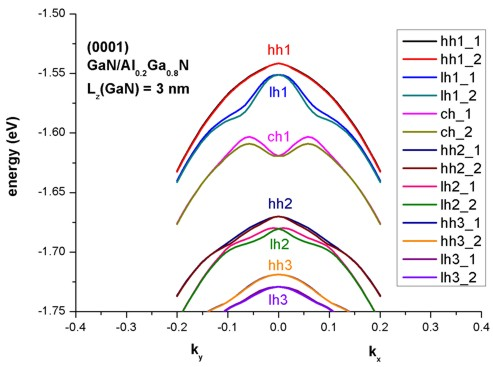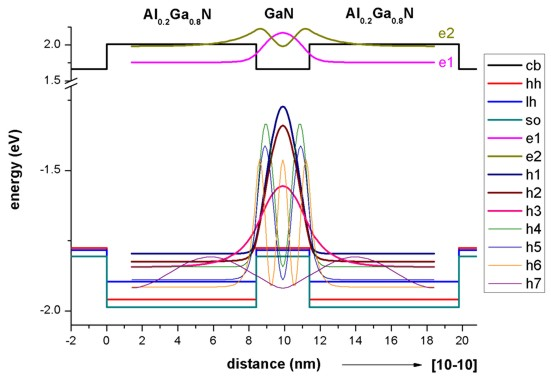nextnano.com  GUI: nextnanomat  Tool: nextnano++  Tool: nextnano³  Tool: nextnano.NEGF  Download | Search | Copyright | News | Publications  * password protected nextnano³ software1D GaN/AlGaN QW dispersion

# nextnano3 - Tutorial

## k.p dispersion of an unstrained GaN QW embedded between strained AlGaN layers

Author: Stefan Birner

If you want to obtain the input files that are used within this tutorial, please contact stefan.birner@nextnano.de.
```-> 1DGaN_AlGaN_QW_k_zero.in                   ```(along```   ```direction)``` -> 1DGaN_AlGaN_QW_k_parallel.in               ```(along```   ```direction)``` -> 1DGaN_AlGaN_QW_k_zero_10m10.in             ```(along``` [10-10] ```direction)``` -> 1DGaN_AlGaN_QW_k_parallel_10m10.in         ```(along``` [10-10] ```direction)``` -> 1DGaN_AlGaN_QW_k_parallel_10m10_whole.in   ```(along``` [10-10] ```direction)``` ```(whole 2D k|| space)``` ```

## k.p dispersion of an unstrained GaN QW embedded between strained AlGaN layers

This tutorial is based on:

`[Park1]`
Crystal Orientation Effects on Electronic Properties of Wurtzite GaN/AlGaN Quantum Wells with Spontaneous and Piezoelectric Polarization

S.-H. Park
Jpn. J. Appl. Phys. 39, 3478 (2000)

The material parameters are taken from
```[Park2] ```Comparison of zinc-blende and wurtzite GaN semiconductors with spontaneous polarization and piezoelectric field effects
S.-H. Park, S.L. Chuang
J. Appl. Phys. 87, 353 (2000)
except those listed in Table 1 of `[Park1]`.

We note that meanwhile a revised set of material parameters is available. However, in order to reproduce the results of` [Park1] `we used the same parameters as in the above cited papers.

### Calculation of the electron and hole energies and wave functions for k|| = 0.

`-> 1DGaN_AlGaN_QW_k_zero.in`

The structure consists of a 3 nm unstrained GaN quantum well, embedded between 8.4 nm strained Al0.2Ga0.8N barriers. The AlGaN layers are strained with respect to the GaN substrate. The GaN quantum well is assumed to be unstrained.

The structure is modeled as a superlattice (or multi quantum well, MQW), i.e. we apply periodic boundary conditions to the Poisson equation.

The growth direction is along the hexagonal axis, i.e. along .

### Conduction and valence band profile

The following figure shows the conduction and valence (heavy hole, light hole and crystal-field split-off hole) band edges of our structure, including the effects of strain, piezo- and pyroelectricity. The ground state electron and the ground state heavy hole wave functions (psi2) are shown. Due to the built-in piezo- and pyroelectric fields, the electron wave function are shifted to the right and the hole wave function to the left (Quantum Confined Stark Effect, QCSE).### Strain

The strain inside the GaN quantum well layer is zero.
The tensile strain in the Al0.2Ga0.8N barriers has been calculated to be exx = eyy = (asubstrate - a) / a = 0.486 (`[Park1]`: 0.484).

The output of the strain tensor can be found in this file:
`=> strain1/strain_cr1D.dat`

### Piezoelectric polarization

The piezoelectric polarization for the  growth direction is zero inside the GaN QW (because the strain is zero in the QW).
In the Al0.2Ga0.8N barriers, the piezoelectric polarization has been calculated to be 0.0081 C/m2 in agreement with Fig. 1(a) of` [Park1]` for angle theta = 0.
The resulting piezoelectric polarization
- at the Al0.2Ga0.8N/GaN interface is -0.0081 C/m2
- at the GaN/Al0.2Ga0.8N interface is  0.0081 C/m2.

The output of the piezoelectric interface charges can be found in this file:
`=> densities/interface_densitiesD.txt`

### Pyroelectric polarization

The pyroelectric polarization for the  growth direction is -0.029 inside the GaN QW.
In the Al0.2Ga0.8N barriers, the pyroelectric polarization has been calculated to be -0.0394 C/m2.
The resulting pyroelectric polarization
- at the Al0.2Ga0.8N/GaN interface is -0.0104 C/m2
- at the GaN/Al0.2Ga0.8N interface is  0.0104 C/m2.
These results are in excellent agreement with Fig. 1(a) of` [Park1]` for angle theta = 0.

The output of the pyroelectric interface charges can be found in this file:
`=> densities/interface_densitiesD.txt`

### Poisson equation

Solving the Poisson equation with periodic boundary conditions (to mimick the superlattice) leads to the following electric fields:
Inside the GaN QW the electric field has been calculated to be -1.551 MV/cm. `[Park1] `reports an electric field of -1.55 MV/cm inside the QW.
The electric field in the AlGaN barrier has been found to be 0.554 MV/cm.

The output of the electrostatic potential (units [V]) and the electric field (units [kV/cm]) can be found in these files:
```=> band_structure/potential1D.dat => band_structure/electric_field1D.dat```

### Schrödinger equation

The following figure shows the electron and hole wave functions (psi2) of the GaN/AlGaN structure for k|| = 0. The heavy and light hole wave functions are very similar in shape.

In agreement with the paper` [Park1]`, we calculated the electron levels within the single-band effective mass approximation and the hole levels within the 6-band k.p approximation.### k|| dispersion: Calculation of the electron and hole energies and wave functions for k|| /= 0.

`-> 1DGaN_AlGaN_QW_k_parallel.in`

The grid has a spacing of 0.1 nm leading to a sparse matrix of dimension 1050 which has to be solved for each k|| point for the eigenvalues (and wave functions).

We chose as input:
```  num-kp-parallel = 1849   ! ```This corresponds to``` 1849 ```k|| points in the 2D (kx,ky) plane, i.e. (2 * 21 + 1) * (2 * 21 + 1) =` 1849`.

Due to symmetry arguments, we solved the the Schrödinger equation only for the k|| points along the line (kx > 0, ky = 0), i.e. we had to solve the Schrödinger equation 22 times (i.e. to calculate the eigenvalues of a 1050 x 1050 matrix 22 times).

The energy dispersion E(k||) = E(kx,ky) is contained in this file:``` Schroedinger_kp\kpar1D_disp_00_10_hl_6x6kp_ev_min001_ev_max014.dat```Because our quantum well is not symmetric (due to the piezo- and pyroelectric fields!), the eigenvalues for spin up and spin down are not degenerate any more. They are only degenerate at k|| = 0.
This lifting of the so-called Kramers degeneracy in the in-plane dispersion relations is because of the field-induced assymetry. In Fig. 3 (a) of` [Park1] ` only the spin-up eigenstates are plotted because the splitting of the Kramers degeneracy was assumed to be very small.

### [10-10] growth direction (m-plane)

```-> 1DGaN_AlGaN_QW_k_zero_10m10.in       ```(along``` [10-10] ```direction)

If one grows the quantum well along the [10-10] growth direction, then the pyroelectric and piezoelectric fields along the [10-10] direction are zero.
In this case, the quantum well (i.e. the conduction and valence band profile) is symmetric.

The following figure shows the electron and hole wave functions (psi2) of the (10-10)-oriented GaN/AlGaN QW for k|| = 0.
Obviously, the interband transition matrix elements (i.e. the probability for electron-hole transitions) are much larger than for the  growth direction.

In agreement with the paper` [Park1]`, we calculated the electron levels within the single-band effective mass approximation and the hole levels within the 6-band k.p approximation.### k|| dispersion: Calculation of the electron and hole energies and wave functions for k|| /= 0.

```-> 1DGaN_AlGaN_QW_k_parallel_10m10.in   ```(along``` [10-10] ```direction)

Due to the symmetry of the quantum well, we expect degenerate eigenvalues for the in-plane dispersion relation (Kramers degeneracy).
Our results compare well with Fig. 3(c) of` [Park1]`.• Please help us to improve our tutorial! Send comments to``` support [at] nextnano.com```.